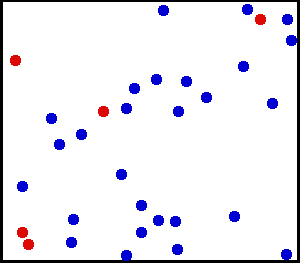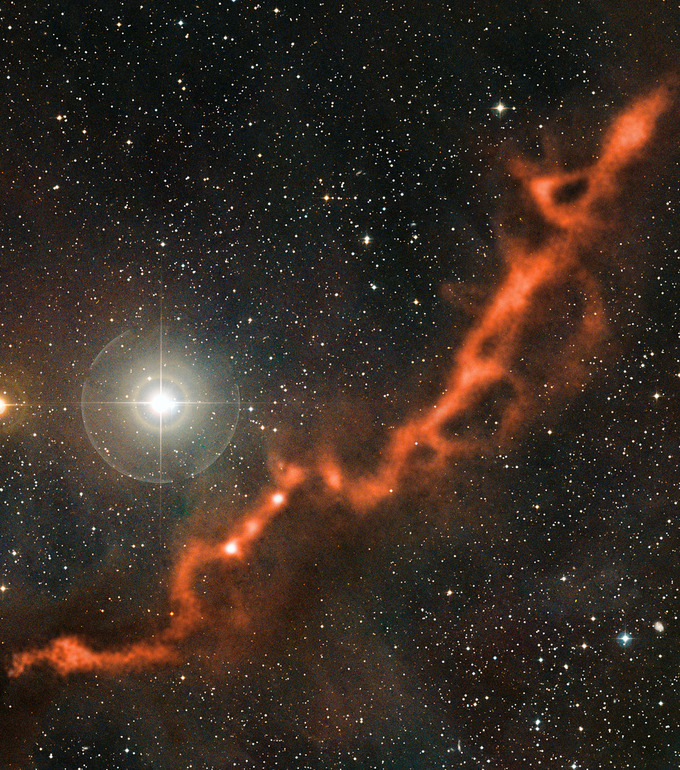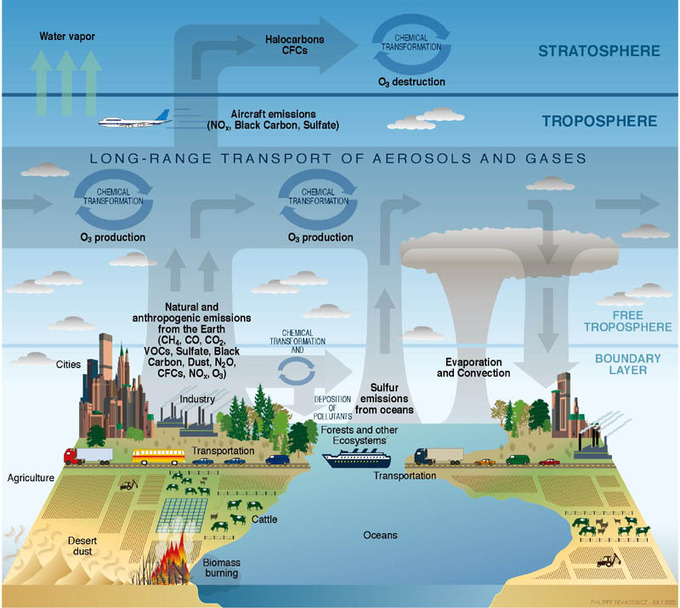The Ideal Gas Equation

The ideal gas equation is given by $PV = nRT$.

Learning Objectives

Apply the ideal gas law to solve problems in chemistry

Key Takeaways

Key Points

• An ideal gas exhibits no attractive forces between particles.
• In the ideal gas equation, both pressure and volume are directly proportional to temperature.

Key Terms

• ideal gas constant: R = 8.3145 J·mol-1·K-1
• ideal gas: a gas whose particles exhibit no attractive interactions whatsoever; at high temperatures and low pressures, gases behave close to ideally
• kinetic energy: the energy possessed by an object because of its motion; in Kinetic Gas Theory, the kinetic energy of gas particles is dependent upon temperature only

Kinetic Theory and Ideal Gases

All gases are modeled on the assumptions put forth by the Kinetic Theory of Matter, which assumes that all matter is made up of particles (i.e. atoms or molecules); there are spaces between these particles, and attractive forces become stronger as the particles converge. Particles are in constant, random motion, and they collide with one another and the walls of the container in which they are enclosed. Each particle has an inherent kinetic energy that is dependent upon temperature only.Translational motion of helium: Real gases do not always behave according to the ideal model under certain conditions, such as high pressure. Here, the size of helium atoms relative to their spacing is shown to scale under 1,950 atmospheres of pressure.

A gas is considered ideal if its particles are so far apart that they do not exert any attractive forces upon one another. In real life, there is no such thing as a truly ideal gas, but at high temperatures and low pressures (conditions in which individual particles will be moving very quickly and be very far apart from one another so that their interaction is almost zero), gases behave close to ideally; this is why the Ideal Gas Law is such a useful approximation.

Ideal Gas Law Introduction: Discusses the ideal gas law PV = nRT, and how you use the different values for R: 0.0821, 8.31, and 62.4.

Ideal Gas Law Equation

The Ideal Gas Equation is given by:

$\displaystyle{PV=nRT}$

The four variables represent four different properties of a gas:

• Pressure (P), often measured in atmospheres (atm), kilopascals (kPa), or millimeters mercury/torr (mm Hg, torr)
• Volume (V), given in liters
• Number of moles of gas (n)
• Temperature of the gas (T) measured in degrees Kelvin (K)

R is the ideal gas constant, which takes on different forms depending on which units are in use. The three most common formulations of R are given by:

$\displaystyle{8.3145\frac{\text{L} \cdot \text{kPa}}{\text{K} \cdot \text{mol}}=0.0821\frac{\text{L} \cdot \text{atm}}{\text{K} \cdot \text{mol}}=62.4\frac{\text{L} \cdot \text{mm Hg}}{K \cdot \text{mol}}}$

Example 1

A 20 L box contains a fixed amount of gas at a temperature of 300 K and 101 kPa of pressure. How many moles of gas are contained in the box?

$PV=nRT$

$\displaystyle{n=\frac{PV}{RT}=\frac{\text{(101 kPa)(20 L)}}{\text{(8.3145 }\text{L} \cdot \text{kPa} \cdot K^{-1} \cdot \text{mol}^{-1}) \cdot \text{300 K}}=\text{0.8 } \text{mol}}$

Example 2

Calculate the number of moles of gas contained within a bouncy house with a volume of 20.63 cubic meters, a temperature of 300 Kelvin, and a pressure of 101 kPa.

$\displaystyle{PV=nRT}$

$\displaystyle{\frac{PV}{RT}=n \cdot n=\frac{101\text{ kPa} \cdot (20.63\text{ cubic meters})}{(8.3143\text{ J/mol}) \cdot K(300K)} \cdot n=835.34\text{ mols}}$

The ideal gas equation enables us to examine the relationship between the non-constant properties of ideal gases (n, P, V, T) as long as three of these properties remain fixed.

For the ideal gas equation, note that the product PV is directly proportional to T. This means that if the gas’ temperature remains constant, pressure or volume can increase as long as the complementary variable decreases; this also means that if the gas’ temperature changes, it may be due in part to a change in the variable of pressure or volume.

The ideal gas equation is a valuable tool that can give a very good approximation of gases at high temperatures and low pressures.

Interactive: Pressure Equilibrium: There are gases on both sides of a moveable barrier (piston), which stays in the same place (more or less) when you run the model because the gas pressure on the piston is in equilibrium. Add purple gas molecules and watch what happens to the piston. Reset the model. Now add yellow gas molecules. What happens to the piston? Try heating or cooling the gas molecules. Explain the change in equilibrium with each change. Which has a greater effect on equilibrium — changing the number of gas molecules or changing the temperature? Why?

Interactive: The Temperature-Pressure Relationship: Explore the relationship between the temperature of a gas and the pressure it exerts on its container.

Density Calculations

A reformulation of the Ideal Gas Equation involving density allows us to evaluate the behaviors of ideal gases of unknown quantity.

Learning Objectives

Apply the reformulated Ideal Gas Equation in your calculations

Key Takeaways

Key Points

• Density calculations allow us to evaluate the behaviors of gases of unknown volume.
• We can determine the density of an ideal gas using knowledge of three properties of the evaluated ideal gas.
• This reformulation of the Ideal Gas Equation relates pressure, density, and temperature of an ideal gas independent of the volume or quantity of gas.

Key Terms

• density: a measure of the amount of matter contained by a given volume

The Ideal Gas Equation in the form $PV=nRT$ is an excellent tool for understanding the relationship between the pressure, volume, amount, and temperature of an ideal gas in a defined environment that can be controlled for constant volume. However, in its most common form, the Ideal Gas Equation is not useful for examining the behavior of gases of undetermined volume, such as the gases in the clouds that surround the stars in our solar system or the atmospheric gases that support life on our planet.Astronomical applications of the Ideal Gas Law: The Taurus Molecular Cloud consists of dust and various gases, including hydrogen and helium. The density form of the Ideal Gas Equation may be of theoretical use when studying such astronomical phenomena as star formation.

To derive a form of the ideal gas equation that has broader applications, we can use calculations that employ the physical property of density.Atmosphere composition: Atmospheric science offers one plausible real-life application of the density form of the ideal gas equation. Earth’s atmosphere is composed of gases that support life—as the image shows, oxygen involved in the water cycle, sulfur emissions from oceans, methane from agriculture, and more. The density form of the Ideal Gas Law enables us to study the behavior of these gases without enclosing them in a container of known volume.

Derivation of the Volume-Independent Ideal Gas Law

We know the Ideal Gas Equation in the form $PV=nRT$. We also know that:

$n=\text{# moles of gas}=\frac{\text{mass of gas (m)}}{\text{molecular weight (M)}}=\frac{m}{M}$

If we substitute $\frac{m}{M}$ for n:

$PV= \frac{m}{M}RT$

Rearranging the above equation, we get:

$\frac{P}{RT}=\frac{m}{MV}$

Now, recall that density is equal to mass divided by volume:

$D=\frac{m}{V}$

The term $\frac{m}{V}$ appears on the right-hand side of the above rearranged Ideal Gas Law. We can substitute in density, D, and get the following:

$\frac{P}{RT}=\frac{D}{M}$

Rearranging in terms of D, we have:

$D=\frac{MP}{RT}$

This derivation of the Ideal Gas Equation allows us to characterize the relationship between the pressure, density, and temperature of the gas sample independent of the volume the gas occupies; it also allows us to determine the density of a gas sample given its pressure and temperature, or determine the molar mass of a gas sample given its density.

Ideal Gas Law Practice Problems with Density – YouTube: Instead of using the regular ideal gas equation, PV=nRT, we use a transformed version (D=PM/RT) to solve a problem with density and molar mass.

Molar Mass of Gas

We can derive a form of the Ideal Gas Equation, PV=nRT, that incorporates the molar mass of the gas (M, $g*mol^{-1}$ ).

Learning Objectives

Apply knowledge of molar mass to the Ideal Gas Law

Key Takeaways

Key Points

• The molecular weight ( molar mass ) of any gas is the mass of one particle of that gas multiplied by Avogadro’s number (6.02 x 1023).
• Knowing the molar mass of an element or compound can help us stoichiometrically balance a reaction equation.
• The average molar mass of a mixture of gases is equal to the sum of the mole fractions of each gas (xi) multiplied by the molar mass (Mi) of that particular gas: $\bar { M} =\sum _{ i }^{ }{ { x }_{ i }{ M }_{ i } }$.

Key Terms

• ideal gas: a hypothetical gas whose molecules exhibit no interaction and undergo elastic collision with each other and with the walls of the container
• molar mass: the mass of one mole of an element or compound
• stoichiometry: the study and calculation of quantitative (measurable) relationships of the reactants and products in chemical reactions (chemical equations)

Molar Mass of Gases and Gas Mixtures

Molar mass (M) is equal to the mass of one mole of a particular element or compound; as such, molar masses are expressed in units of grams per mole (g mol–1) and are often referred to as molecular weights. The molar mass of a particular gas is therefore equal to the mass of a single particle of that gas multiplied by Avogadro’s number (6.02 x 1023 ). To find the molar mass of a mixture of gases, you need to take into account the molar mass of each gas in the mixture, as well as their relative proportion.

The average molar mass of a mixture of gases is equal to the sum of the mole fractions of each gas, multiplied by their respective molar masses:

$\bar { M} =\sum _{ i }^{ }{ { x }_{ i }{ M }_{ i } }$

The molar volumes of all gases are the same when measured at the same temperature and pressure (22.4 L at STP), but the molar masses of different gases will almost always vary.

Calculating Molar Mass using the Ideal Gas Equation

The molar mass of an ideal gas can be determined using yet another derivation of the Ideal Gas Law: $PV=nRT$.

We can write n, number of moles, as follows:

$n=\frac{m}{M}$

where m is the mass of the gas, and M is the molar mass. We can plug this into the Ideal Gas Equation:

$PV=\left(\frac{m}{M}\right)RT$

Rearranging, we get:

$\frac{PV}{RT}=\frac{m}{M}$

Finally, putting the equation in terms of molar mass, we have:

$M=\frac{mRT}{PV}$

This derivation of the Ideal Gas Equation is useful in determining the molar mass of an unknown gas.

Example

• An unknown gas with a mass of 205 g occupies a volume of 20.0 L at standard temperature and pressure. What is the molar mass of the gas?
• $M=\frac{mRT}{PV}$

$M=\frac{(205)(0.0821)(273)}{(1.0)(20.0)}=230\frac{g}{mol}$

Ideal Gas Law Practice Problems with Molar Mass – YouTube: How to set up and solve ideal gas law problems that involve molar mass and converting between grams and moles.